Courses
Courses for Kids
Free study material
Free LIVE classes
More

# Representing Real Numbers on the Number LineLIVE
Join Vedantu’s FREE Mastercalss

## An Introduction to the Real Number Line

A number line is a horizontal line used to represent integers in Mathematics. Positive and negative integers are both represented on the number line, which is endlessly long in both directions. Real numbers can be represented on a number line for the purposes of comparison and ordering.

Real numbers can be represented visually by assigning them to specific points on a line using a real number line, also known as a number line. This article will go into great detail on how to express the real number on a number line.

## Real Numbers Definition

$Q$$Q'$ are the components of real numbers. $R$ represents an array of real numbers. Real numbers are a category that includes all types of numbers, including whole numbers, natural numbers, integers, decimals, and rational and irrational numbers among others. As a result, $R = Q + Q\prime$ can be written.

Real numbers are shown in the following graph: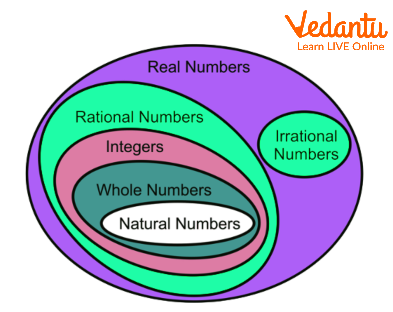Real Numbers

## Real Number Line

Every real number can be represented by a different point on the number line since we know that real numbers can be either rational or irrational. A real number line, also known as a number line, represents real numbers on the line with distinct points assigned to each of the numbers.

• A coordinate is a point that is related to real numbers.

• The origin of the number line is referred to as the number $0$.

• The left side of the origin represents all the negative numbers or integers, while the right side represents all the positive numbers or integers.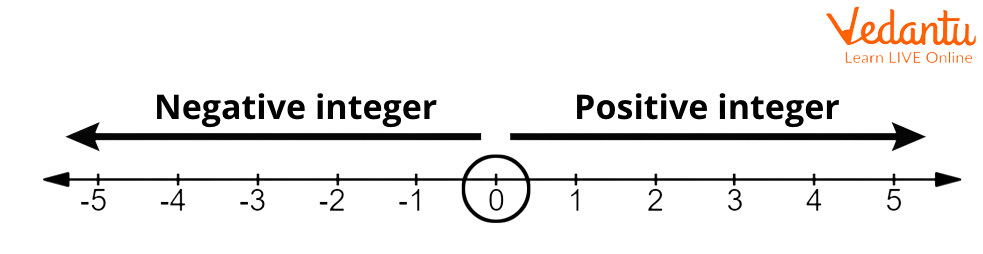Representation of Real Numbers on a Number Line

## How to Represent Real Numbers on the Number Line?

The steps that are taken to represent real numbers on the number line are as follows:

• Step 1: Mark a point anywhere along a horizontal straight line with arrows at both ends. The origin is referred to as zero.

• Step 2: Find real numbers on both sides of the origin spaced equally apart, and give them scale labels.

• Step 3: Numbers that are positive are on the right side of the origin, and whole numbers that are negative are on the left.

• Step 4: By finding the specific point on the number line, you may quickly mark whole numbers, natural numbers, and integers. To represent large numbers efficiently, the number line's scale can be increased. Suppose a specified point is a big number, like $120$. In order to designate $120$, we can choose $1$ unit as $20$, which requires us to move $6$ steps to the right of the origin.

• Step 5: Mark both rational and irrational values on the number line after converting them to decimal counterparts. Sometimes it is impossible to mark a number exactly where it should be. In this situation, we can apply the technique of successive magnification, using the magnifying glass to see the numbers on the number line.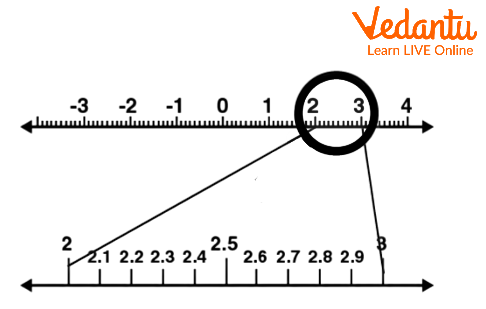Real Numbers on a Number Line

## Integers on a Number Line

Integers are represented on a number line as a combination of both positive and negative values. On the number line, integers rise from left to right. Between positive and negative integers, $0$ resides in the middle. Negative numbers are $- 1$ , $- 2$ , $- 3$ , etc., while positive numbers are $1$ , $2$ , $3$ , etc.

The number line below displays the integers.Integers on Number Line

## Rational Numbers on the Number Line

Numbers in the form of $\dfrac{p}{q}$ where $p$ and $q$ are numerators and denominators, respectively, having integer values are considered rational numbers on a number line. To represent the rational numbers, we divide the two adjacent integers into $n$ parts.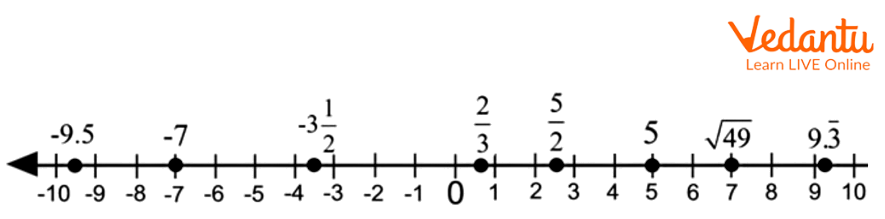Rational Numbers on Number Line

## Decimals on Number Line

To represent decimal numbers, we divide pairs of successive integers into tenths $(10)$, hundredths $(100)$, and so forth.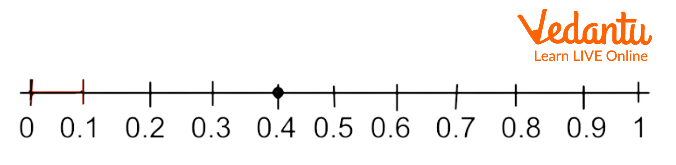Decimals on number line

## Number Line with Irrational Numbers

Real numbers that cannot be expressed as simple fractions are referred to as irrational numbers. They cannot be formulated as a ratio.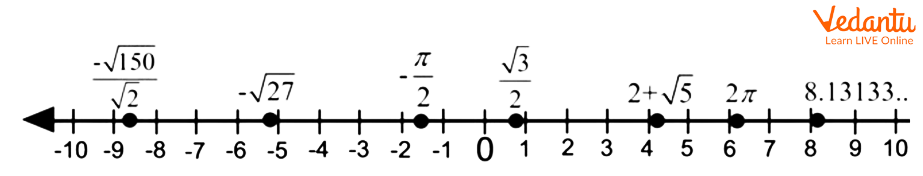Irrational numbers on number line

## Ordered Real Numbers on a Number Line

A number line can also be used for comparing any given real numbers. Always, the larger numbers are to the right of the origin, while the smaller numbers are to the left. Although we are aware that $19$ is bigger than $10$, the sequence is different for negative real numbers. For instance, since negative integers start from the left of the origin, $- 2$ is greater than $- 6$.

It becomes easier to compare numbers after we graph each one on a number line. Real numbers are compared using symbols like less than $( < )$ , greater than $( > )$ , and equal to $( = )$ .

The example of comparing two real numbers with the formula shown above is depicted in the image below.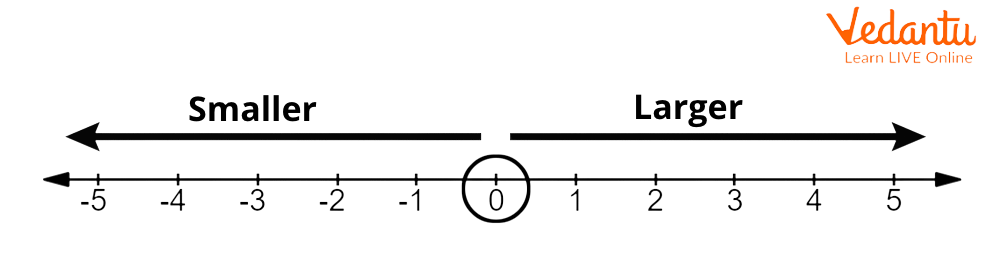Ordering Real Numbers on a Number Line

## A Number Line with Opposing Real Numbers

On a number line, a negative real number is the opposite of a positive real number, and a positive real number is the opposite of a negative real number, i.e., the opposite of $- 8$ is $8$, which is the opposite of $- 8$. According to their signs, the numbers are plotted at the same distance from the original but in the opposite direction. As an illustration, $8$ is the reciprocal of $- 8$. As a guide, take a look at the illustration below: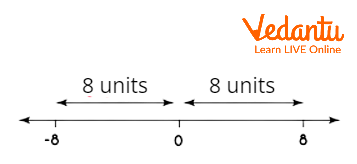Number Line with Opposite Rreal Numbers

## Real Numbers' Absolute Values on a Number Line

The distance between the origin and the real number's graph on the number line is known as the absolute value of a real number, represented by the symbol $\left| x \right|$. It is always positive because it is a distance. As an illustration, $\left| { - 3} \right| = 3$ and $\left| 3 \right| = 3$ . View the illustration below as a guide: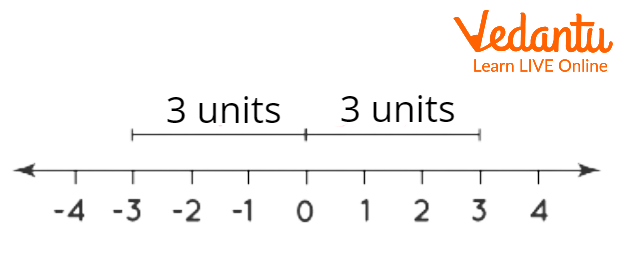Absolute real values on a number line

## Real Number Line Examples

Q.1. Express $\sqrt {2}$ on a number line.

Solution. Consider the irrational number $\sqrt {2}$.

• Mark the origin and other integers on a number line.

• Use a compass to determine the distance between $0$ and $1$.

• Draw a parallel to 1 that has the same length as the distance between $0$ and $1$

• Connect the opposite end of the new line—the perpendicular end—to the origin. You will receive the indicated figure.

• The number $1$ on the number line, the perpendicular end, and the name origin are all A.

• $\sqrt {2}$ is the distance between A and C.

• Calculate the distance between A and C using a compass.

• Set the compass at A and indicate on the number line the same distance between A and C.

• You also reach the $\sqrt {2}$ point at D.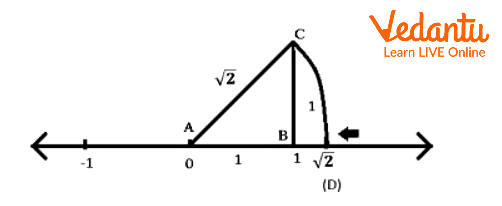Irrational Number $\sqrt {2}$ on a Number Line

Q.2. On a number line, show that $x < 5$.

Solution. The procedures listed below will help you represent $x < 5$ on a number line.

• Draw a number line, mark $0$, and then draw equal distances to the right and left.

• The value of $x$ is less than $5$ in the inequality $x < 5$. We must then move to the left of $5$.

• Create a black empty circle on number five and shade the line from number five to the left until it reaches the arrow as shown in the illustration. In order to demonstrate that point $5$ is not included, the circle at that location will be left unfilled. This proves that $x < 5$.

• On the number line, this mark will reflect the necessary inequality.

## Practice Questions

1. Add two numbers $5$ and $8$ using the number line.

Ans: $13$

2. Express the subtraction of $7$ from $14$ on a number line.

Ans: $7$

## Summary

In this topic, we studied what real numbers are as well as their classifications. We discussed what a number line is and how to use it to represent real numbers. We have also discussed how to express real numbers on a number line using examples. There are also examples with solutions to help you understand the topic better.

Last updated date: 02nd Oct 2023
Total views: 95.4k
Views today: 1.95k

## FAQs on Representing Real Numbers on the Number Line

1. How are real numbers categorised?

Rational and irrational numbers are the two broad categories into which real numbers can be divided. Integers, whole numbers, natural numbers, ratios, and terminating and repeating decimals are the key ideas under the category of rational numbers. Rational numbers include things like $- 5$ , $9.666$ , $0$ , $11$ and so on. Non-terminating and non-repeating decimals are ideas categorised under irrational numbers. The square root of $8$, the square root of $3$, $\pi$, and others are a few instances. $R$ is a representation of the set of real numbers.

2. What are the different types of number lines?

The different types of number lines are mentioned below:

• Real number line

• A circular number line and

• Imaginary number lines

3. Which numbers are used within number lines?

A number line is a graphical representation of numerical data that shows fractions, integers, and whole numbers in a regular pattern along a straight, horizontal line. A number line can be used as a tool for operations like addition, subtraction, and more as well as for comparing and organising numbers.